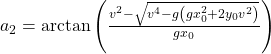# Any way to calculate bullet drop through gravity

I’ve been making a sort of gun system where when you shoot your bullet flies down but I noticed that letting roblox gravity do the thing is the most good looking option but that makes another problem, how can I calculate the bullet drop if all I’m using is roblox’s gravity?

1 Like

Things fall at `workspace.Gravity` studs per second, per second.

What do you mean by bullet drop?

How fast the bullet drops when in air

Then yeah, `workspace.Gravity` (default 196.2) studs/sec/sec.

This is correct but for realism, bullets won’t drop like player characters/physic affected parts do. You might want to cut gravity in half or so.

Ignoring some weird air effect, the fired bullet would fall at the same speed as everything else.

1 Like

You see what I am trying to do is give player the stats about the gun i.e How fast bullet travels and the distance of object and then be able to calculate the angle to aim at to hit the object

I made this desmos a while ago that does some of these calculations: Projectile Motion Calculation

You can change the velocity/gravity with the sliders on the left, and you can drag the target point around. Maybe the angle calculations will be useful to you.

tl;dr:Where v is the launch velocity, g is gravity, and (x_0, y_0) is the target.

I’ll leave it as an excercise for the reader to turn it into Lua4 Likes

You can obviously make some big simplifications if you assume everything is on the x axis

There are many equations from the Projectile motion - Wikipedia page (that’s probably where I stole the above one from in the first place)

There’s also Range of a projectile - Wikipedia

Also some quick math: if you are firing the gun at 0 degrees, from a height h, at a horizontal velocity v, you can pretty easily figure out the distance the bullet will travel before it hits the ground: Bullet range

In lua:

``````local g = workspace.Gravity
local h = 3.5 -- about robloxian armpit height
local v = 1000 -- studs/sec
local dist = v * math.sqrt(2*g*h) / g
``````

You could also use that to figure out the ‘effective range’ of a gun by setting `h` to however much you’re willing to let the bullet drop before it’s considered ineffective.

So like a 1000 stud/sec bullet would travel about 100 studs before it dropped 1 stud.

3 Likes